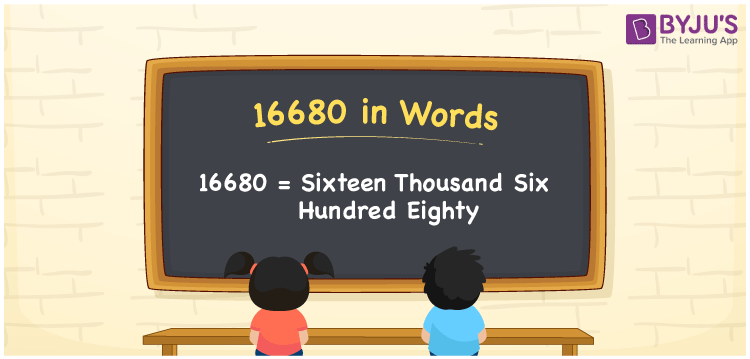# 16680 in Words

16680 in words is written as sixteen thousand six hundred eighty. For example, Rohit gave Rs. 16680 to his friend. It can also be written as “Rohit gave Rs. Sixteen thousand six hundred eighty to his friend”. This article will help you write the number 16680 in words using the place values of the number.

 16680 in Words: Sixteen Thousand Six Hundred Eighty. Sixteen Thousand Six Hundred Eighty in Numerical Form: 16680.

## 16680 in English Words## How to Write 16680 in Words?

Since the number 16680 has five digits, its place value table up to 5 digits are given below.

 Ten-thousands Thousands Hundreds Tens Ones 1 6 6 8 0

The expanded form of 16680 is as follows:

= 1 × Ten thousand + 6 × Thousand + 6 × Hundred + 8 × Ten + 0 × One

= 1 × 10000 + 6 × 1000 + 6 × 100 + 8 × 10 + 0 × 1

= 10000 + 6000 + 600 + 80

= 16680

= Sixteen thousand six hundred eighty

Hence, 16680 in words is sixteen thousand six hundred eighty.

16680 in words – Sixteen thousand six hundred eighty

Is 16680 an odd number? – No

Is 16680 an even number? – Yes

Is 16680 a perfect square number? – No

Is 16680 a perfect cube number? – No

Is 16680 a prime number? – No

Is 16680 a composite number? – Yes

## Frequently Asked Questions on 16680 in Words

Q1

### How to spell 16680?

16680 in words is sixteen thousand six hundred eighty.

Q2

### Simplify 16000 + 680, and express it in words.

Simplifying 16000 + 680, we get 16680. Hence, 16680 in words is sixteen thousand six hundred eighty.

Q3

### Is 16680 a composite number?

Yes, 16680 is a composite number.